Author: Oscar Cronquist Article last updated on May 05, 2022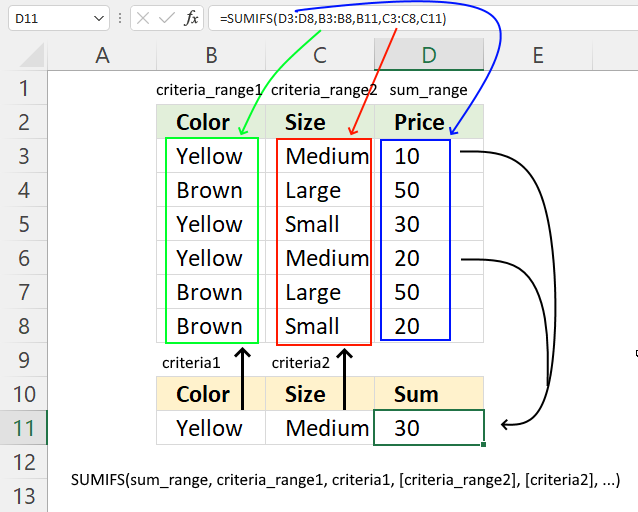The SUMIFS function calculates a total based on multiple criteria, it has been available in Excel since version 2010. I recommend the SUMPRODUCT function if you use an earlier Excel version than 2010.

The SUMIFS function in cell D11 adds numbers from column D based on criteria applied to columns B and C.

=SUMIFS(D3:D8,B3:B8,B11,C3:C8,C11)

This article explains how to use the SUMIFS function in great detail.

## 1. SUMIFS Function Syntax

SUMIFS(sum_range, criteria_range1, criteria1, [criteria_range2], [criteria2], ...)

## 2. SUMIFS Function Arguments

 sum_range Required. A cell reference to a cell range whose numbers you want to sum. criteria_range1 Required. The cell range you want to test Criteria1 for. criteria1 Required.  The condition you want to use applied to criteria_range1 to sum the corresponding cells in sum_range [criteria_range2] Optional.  Up to 127 additional arguments. [criteria2] Optional.  Up to 127 additional arguments.

## 3. SUMIFS function greater than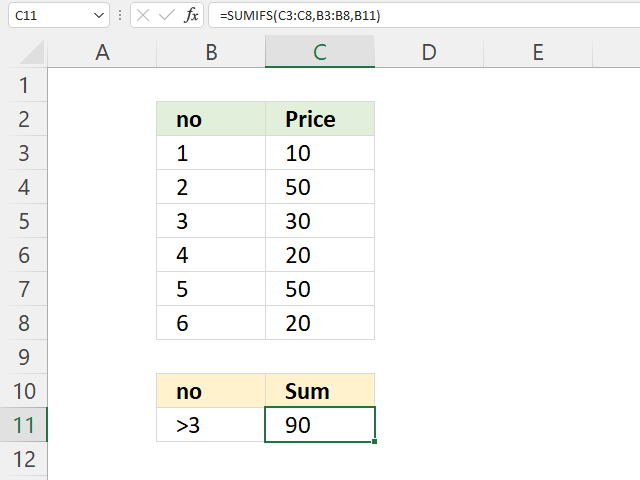The image above shows the SUMIFS function cell C11, it adds numbers from C3:C8 if the corresponding number in cells B3:B8 is larger than 3. Cell B11 specifies the condition, notice the larger than character combined with number 3.

Cells B6, B7,  and B8 all contain numbers higher than 3. The corresponding numbers are in cells C6, C7, and C8. These are 20 + 50 + 20 equals 90.

Formula in cell C11:

=SUMIFS(C3:C8, B3:B8, B11)

### 3.1 Explaining formula

#### Step 1 - Populate arguments

SUMIFS(sum_range, criteria_range1, criteria1, [criteria_range2], [criteria2], ...)

becomes

SUMIFS(C3:C8, B3:B8, B11)

#### Step 2 - Evaluate SUMIFS function

SUMIFS(C3:C8, B3:B8, B11)

becomes

SUMIFS({10; 50; 30; 20; 50; 20},{1; 2; 3; 4; 5; 6},">3")

and returns 90.

4, 5, and 6 are larger than three. The corresponding numbers on the same rows are 20, 50, and 20.

20 + 50 + 20 equals 90.

## 4. SUMIFS function not equal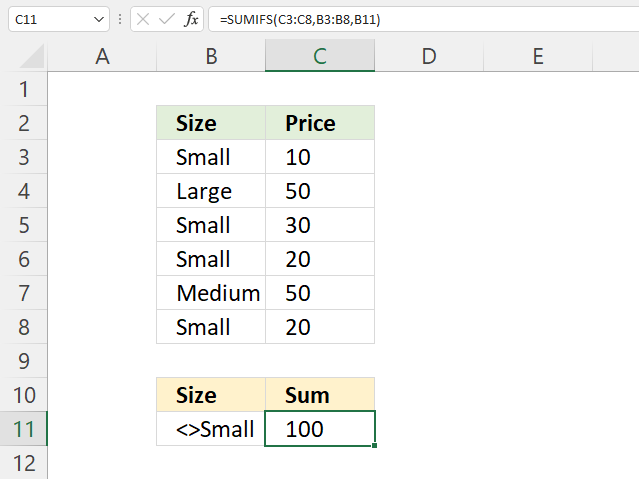The image above demonstrates a SUMIFS function in cell C11 that adds numbers from C3:C8 if the corresponding value on the same row in cells B3:B8 are not equal to "Small".

Cell B11 specifies the condition, cells B7 and B4 meet the condition and the corresponding numbers are 50 and 50. The total is 100, 50 + 50 equals 100.

Formula in cell C11:

=SUMIFS(C3:C8, B3:B8, B11)

### 4.1 Explaining formula

#### Step 1 - Populate arguments

SUMIFS(sum_range, criteria_range1, criteria1, [criteria_range2], [criteria2], ...)

becomes

SUMIFS(C3:C8, B3:B8, B11)

#### Step 2 - Evaluate SUMIFS function

SUMIFS(C3:C8, B3:B8, B11)

becomes

SUMIFS({10; 50; 30; 20; 50; 20}, {"Small"; "Large"; "Small"; "Small"; "Medium"; "Small"}, "<>Small")

and returns 100. "Large" and "Medium" are not equal to Small, the corresponding values on the same rows are 50 and 50.

50 + 50 equals 100.

## 5. SUMIFS function multiple criteria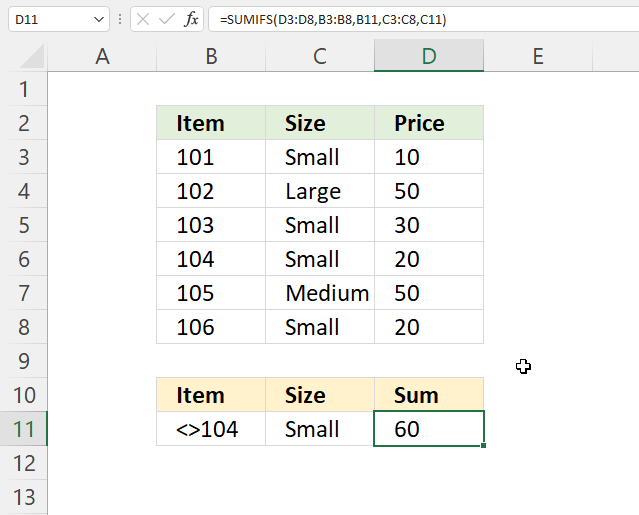This section describes how to use multiple conditions in the SUMFIS function. The image above demonstrates an example with two conditions, each condition applies to a separate column.

Formula in cell D11:

=SUMIFS(D3:D8, B3:B8, B11, C3:C8, C11)

### 5. Explaining formula

#### Step 1 - Populate arguments

SUMIFS(sum_range, criteria_range1, criteria1, [criteria_range2], [criteria2], ...)

becomes

SUMIFS(D3:D8, B3:B8, B11, C3:C8, C11)

#### Step 2 - Evaluate SUMIFS function

SUMIFS(D3:D8, B3:B8, B11, C3:C8, C11)

becomes

SUMIFS({10; 50; 30; 20; 50; 20},{101; 102; 103; 104; 105; 106},"<>104",{"Small"; "Large"; "Small"; "Small"; "Medium"; "Small"},"Small")

and returns 60. 10 + 30 + 20 equals 60.

## 6. SUMIFS function - OR logic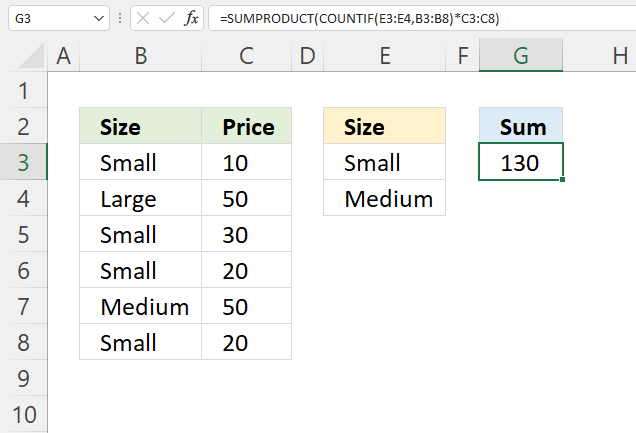This example demonstrates how to sum numbers using OR logic, however, I recommend using the SUMPRODUCT function in this case. The SUMIFS function won't allow me to use functions, only cell references, in the second argument.

The formula in cell G3 uses two conditions, if any of the conditions match a value in B3:B8 the corresponding number on the same row in C3:C8 is added to a total. Cells B3, B5, B6, B7, and B8 match one of the conditions, the corresponding cells on the same rows are B3, B5, B6, B7, and B8. 10 + 30 + 20 + 50 + 20 equals 130.

Formula in cell G3:

=SUMPRODUCT(COUNTIF(E3:E4, B3:B8)*C3:C8)

### 6.1 Explaining formula

#### Step 1 - Identify cells equal to criteria

The COUNTIF function calculates the number of cells that is equal to a condition.

COUNTIF(rangecriteria)

COUNTIF(E3:E4, B3:B8)

becomes

COUNTIF({"Small"; "Medium"}, {"Small"; "Large"; "Small"; "Small"; "Medium"; "Small"})

and returns {1; 0; 1; 1; 1; 1}.

#### Step 2 - Multiply array with numbers

The asterisk character lets you multiply numbers in Excel, this works fin with arrays as well.

COUNTIF(E3:E4, B3:B8)*C3:C8

becomes

{1; 0; 1; 1; 1; 1}*{10; 50; 30; 20; 50; 20}

and returns {10; 0; 30; 20; 50; 20}.

#### Step 2 - Add numbers and return a total

The SUMPRODUCT function calculates the product of corresponding values and then returns the sum of each multiplication.

SUMPRODUCT(array1, [array2], ...)

SUMPRODUCT(COUNTIF(E3:E4, B3:B8)*C3:C8)

becomes

SUMPRODUCT({10; 0; 30; 20; 50; 20})

and returns 130 in cell 130.

## 7. SUMIFS Function example using partial match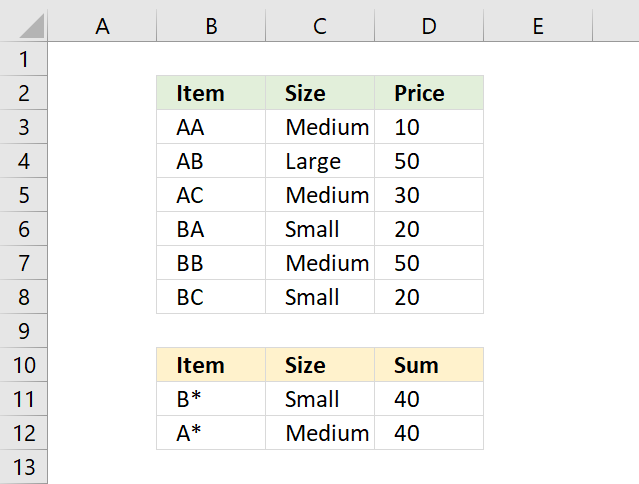The SUMIFS function in cell D11 sums all corresponding values that begin with B in column B and is Small in column C.

=SUMIFS(D3:D8, B3:B8, B11, C3:C8, C11)

You can use wildcard characters like:

• * (asterisk) - Matches any length of characters also 0 (zero)
• ? (question mark) - matches any single character

### 7.1 Explaining formula

#### Step 1 - Populate arguments

SUMIFS(sum_range, criteria_range1, criteria1, [criteria_range2], [criteria2], ...)

becomes

SUMIFS(D3:D8, B3:B8, B11, C3:C8, C11)

#### Step 2- Evaluate SUMIFS function

SUMIFS(D3:D8, B3:B8, B11, C3:C8, C11)

becomes

SUMIFS({10; 50; 30; 20; 50; 20},{"AA"; "AB"; "AC"; "BA"; "BB"; "BC"},"B*",{"Medium"; "Large"; "Medium"; "Small"; "Medium"; "Small"},"Small")

and returns 40 in cell D11. 20 + 20 equals 40.

## 8. How to use logical operators in the SUMIFS function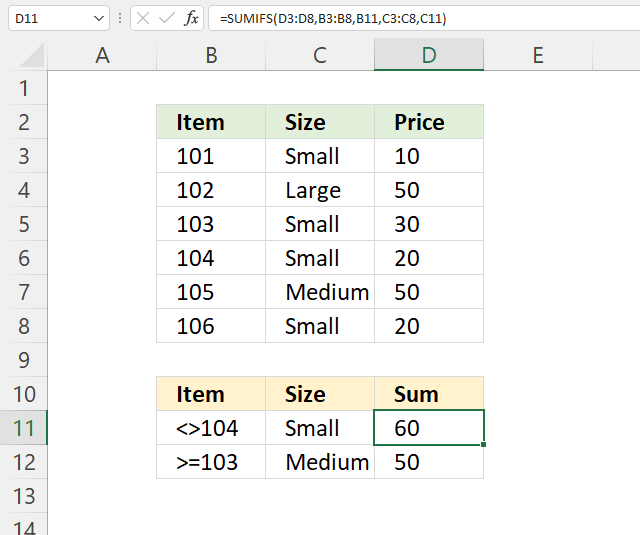The formula in cell D11 sums numbers in column D based on numbers less than 104 in column B and Small in column C.

=SUMIFS(D3:D8, B3:B8, B11, C3:C8, C11)

You are also allowed to use logical operators like:

• > larger than
• < smaller than
• = equal to
• <> not equal to
• =>larger than or equal to
• =<less than or equal to

### 8.1 Explaining formula

#### Step 1 - Populate arguments

SUMIFS(sum_range, criteria_range1, criteria1, [criteria_range2], [criteria2], ...)

becomes

SUMIFS(D3:D8, B3:B8, B11, C3:C8, C11)

#### Step 2- Evaluate SUMIFS function

SUMIFS(D3:D8, B3:B8, B11, C3:C8, C11)

becomes

SUMIFS({10; 50; 30; 20; 50; 20},{101; 102; 103; 104; 105; 106},"<>104",{"Small"; "Large"; "Small"; "Small"; "Medium"; "Small"},"Small")

and returns 60. 10 + 30 + 20 equals 60.

## 9. How do I sum values between n-th weekday this month and n-th weekday next month?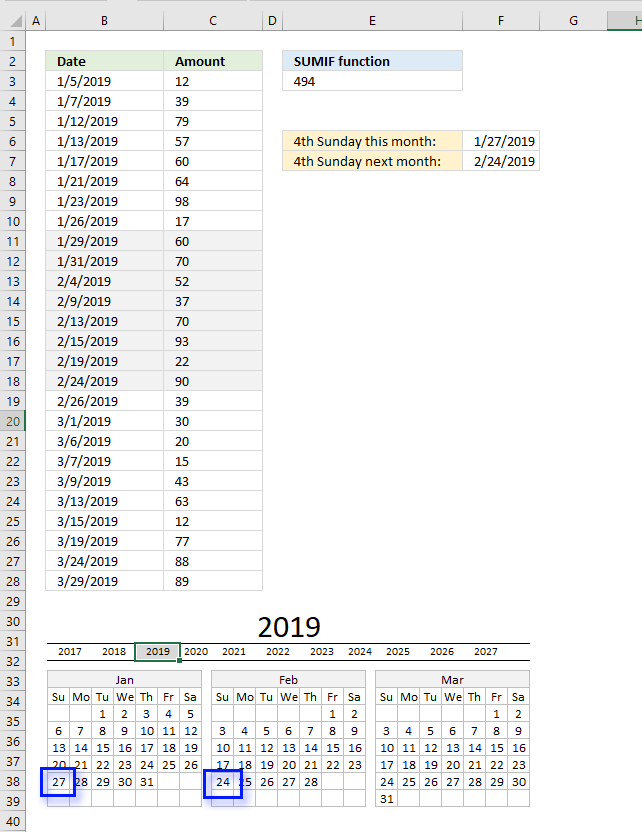I have this formula

(=SUMIFS(\$C\$14:\$C\$1000,\$A\$14:\$A\$1000,">="&DATE(\$A\$1,8,1),\$A\$14:\$A\$1000,"<"&DATE(\$A\$1,9,1),\$F\$14:\$F\$1000,\$AA1))

which works but I want it to show from the 4th Sunday of a month to the 4th Sunday of the next month.

Formula in cell E3:

=SUMIFS(C3:C28, B3:B28, ">="&B3+(7-WEEKDAY(B3, 2))+21, B3:B28, "<="&DATE(YEAR(B3), MONTH(B3)+1, 1)+(7-WEEKDAY(DATE(YEAR(B3), MONTH(B3)+1, 1), 2))+21)

### 9.1 Explaining formula in cell E3

#### Step 1 - Calculate 4-th sunday this month

To calculate the fourth sunday we must first calculate the first Sunday in current month. Cell B3 contains this date 1/5/2019.

WEEKDAY(B3, 2) returns a number representing the weekday. 1 is Monday, 2 is Tuesday and so on.. 7 is Sunday.

WEEKDAY(B3, 2)

becomes

WEEKDAY(1/5/2019, 2)

returns 6.

7 minus 6 equals 1. We must add 1 to the date in cell B3 to get the first Sunday in that month. (This calculation works only if the date in cell B3 is less or equal to the date of the first Sunday in that month.)

B3+(7-WEEKDAY(B3, 2))+21

becomes

1/5/2019+(7-WEEKDAY(B3, 2))+21

becomes

1/5/2019+1+21

To get the fourth Sunday we add 21 to the date of the first Sunday.

1/5/2019+1+21 equals 1/27/2019.

#### Step 2 - Calculate 4-th sunday next month

To get the first day of the next month we need to calculate the first date of this month and then add 1 to the month.

DATE(YEAR(B3), MONTH(B3)+1, 1)

becomes

DATE(2019, 1+1, 1)

becomes

DATE(2019, 2, 1)

and returns 2/1/2019. This date is also used to calculate the fourth Sunday.

DATE(YEAR(B3), MONTH(B3)+1, 1)+(7-WEEKDAY(DATE(YEAR(B3), MONTH(B3)+1, 1), 2))+21

becomes

2/1/2019+(7-5)+21

becomes

2/1/2019+23 equals 2/24/2019.

#### Step 3 - Build SUMIFS function

The SUMIFS function allows you to add values based on conditions.

SUMIFS(C3:C28, B3:B28, ">="&B3+(7-WEEKDAY(B3, 2))+21, B3:B28, "<="&DATE(YEAR(B3), MONTH(B3)+1, 1)+(7-WEEKDAY(DATE(YEAR(B3), MONTH(B3)+1, 1), 2))+21)

becomes

SUMIFS(C3:C28, B3:B28, ">="&1/27/2019, B3:B28, "<="&2/24/2019)

and returns 494.

60+70+52+37+70+93+22+90 = 494